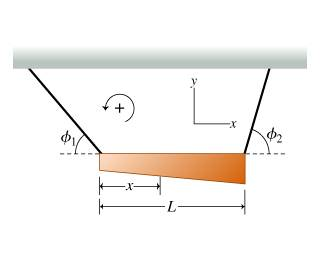## Homework Statement

A nonuniform, horizontal bar of mass m is supported by two massless wires against gravity. The left wire makes an angle ϕ1 with the horizontal, and the right wire makes an angle ϕ2. The bar has length L.

Find the position of the center of mass of the bar, x, measured from the bar's left end.net torque = 0

## The Attempt at a Solution

this is how i did it, the answer was wrong :(
http://i.imgur.com/LwxnukY.jpg
and all answers i found online was L/((tanø2/tanø1)+1) which is incorrect.
help please, thank you very much

Last edited:

Seems like you forgot to ask a question.

Seems like you forgot to ask a question.

my bad, I edited the question. would you please tell me how to do it? :P thank you!

BiGyElLoWhAt
Gold Member
check your first line of equations, look at the units and tell me if that's a legitimate statement. I'm talking about the sum x torque equation. I haven't looked too far into the question yet, but I think that may be a good chunk of your problem
hint: (where's the center of mass in your equation?!?!? how can you solve for something that isn't there?)
you need some x's and some L-x's and junks and stuffs and things...

check your first line of equations, look at the units and tell me if that's a legitimate statement. I'm talking about the sum x torque equation. I haven't looked too far into the question yet, but I think that may be a good chunk of your problem
hint: (where's the center of mass in your equation?!?!? how can you solve for something that isn't there?)
you need some x's and some L-x's and junks and stuffs and things...

I guess i should write sum force x, but isn't the equation itself correct?

for the second equation, i setted the torque around center of mass to be 0; then calculated for x which is the distance from the left end to the center of mass.

BiGyElLoWhAt
Gold Member
I'm not sure if i would say that it's "incorrect", just not useful. It looks like to me you need to use $r X F = T$
real quick, are you supposed to find a 1d coordinate for the CoM, or (x,y) coordinate? the second ones more complex.
if it's 1d, I'd start with $xT_{1} sin(\Phi_{1}) + (L-x)T_{2}sin(\Phi_{2}) = 0 ∴ xT_{2} sin(\Phi_{1}) = -(L-x)T_{2}sin(\Phi_{2})$
then solve for x:
$x T_{1}sin(\Phi_{1}) = xT_{2} sin(\Phi_{2}) - LT_{2} sin(\Phi_{2}) ∴$
$x [T_{1} sin(\Phi_{1})- T_{2}sin(\Phi_{2})] = -LT_{2} sin(\Phi_{2}) ∴$
$x = \frac{-LT_{2} sin(\Phi_{2})}{T_{1}sin(\Phi_{1})-T_{2}sin(\Phi_{2})}$

Try that out

Last edited:
•1 person
BiGyElLoWhAt
Gold Member
if it's 1d, I'd start with $xT_{1} sin(\Phi_{1}) + (L-x)T_{2}sin(\Phi_{2}) = 0 ∴ xT_{2} sin(\Phi_{1}) = -(L-x)T_{2}sin(\Phi_{2})$
then solve for x:
$x T_{1}sin(\Phi_{1}) = xT_{2} sin(\Phi_{2}) - LT_{2} sin(\Phi_{2}) ∴$
$x [T_{1} sin(\Phi_{1})- T_{2}sin(\Phi_{2})] = -LT_{2} sin(\Phi_{2}) ∴$
$x = \frac{-LT_{2} sin(\Phi_{2})}{T_{1}sin(\Phi_{1})-T_{2}sin(\Phi_{2})}$

I guess i should clarify, T's are tensions, so your $F_{1}$ and $F_{2}$

BiGyElLoWhAt
Gold Member
then you can solve for T1 and T2, I'll let you do that, mostly because I'm feeling lazy lol, I'm not sure what all you have to do.

then you can solve for T1 and T2, I'll let you do that, mostly because I'm feeling lazy lol, I'm not sure what all you have to do.

thank you so much
after reading your reply i found out that i made a foolish calculation mistake. The correct answer should be x = (sinø_2 L) / (tan ø_1 cos ø_2 + sinø_2), i was right, but i simplified it incorrectly, now i can't figure out how to simplify this is expression in terms of tangents :(

BiGyElLoWhAt
Gold Member
If I'm reading your solution correctly, I'm not sure that it does, unless you reeeaaalllyyy want those tangents, you could probably do some multiply by 1. Tan^2 + 1= sec^2
Sin * sec = tan ... plus I'm not sure where you got that tan in the first place

haruspex